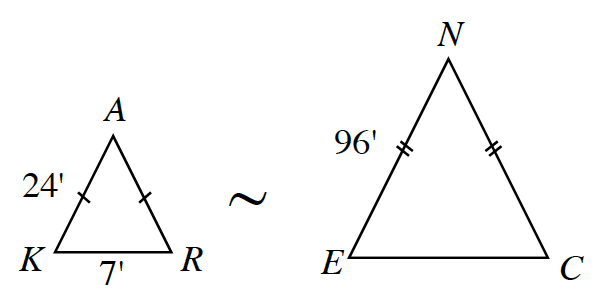### Home > CCG > Chapter 4 > Lesson 4.1.2 > Problem4-18

4-18.The triangles shown at right are similar.

1. What is the ratio of side length $NE$ to side length $AK$?

$\frac{NE}{AK}=\frac{96}{24}=4$

2. Use a ratio to compare the perimeters of $ΔENC$ and $ΔKAR$. How is the perimeter ratio related to the side length ratio?

In part (a), you found that the zoom factor between the two triangles is $4$.
Now use this information to find the perimeters of each triangle, then find the ratio between them.

3. If you have not already done so, find the length of $\overline{EC}$.